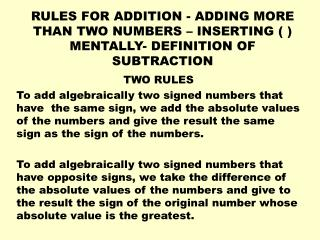DownloadDownload PresentationRULES FOR ADDITION - ADDING MORE THAN TWO NUMBERS – INSERTING ( ) MENTALLY- DEFINITION OF SUBTRACTION

# RULES FOR ADDITION - ADDING MORE THAN TWO NUMBERS – INSERTING ( ) MENTALLY- DEFINITION OF SUBTRACTION

Télécharger la présentation## RULES FOR ADDITION - ADDING MORE THAN TWO NUMBERS – INSERTING ( ) MENTALLY- DEFINITION OF SUBTRACTION

- - - - - - - - - - - - - - - - - - - - - - - - - - - E N D - - - - - - - - - - - - - - - - - - - - - - - - - - -
##### Presentation Transcript

1. RULES FOR ADDITION - ADDING MORE THAN TWO NUMBERS – INSERTING ( ) MENTALLY- DEFINITION OF SUBTRACTION TWO RULES To add algebraically two signed numbers that have the same sign, we add the absolute values of the numbers and give the result the same sign as the sign of the numbers. To add algebraically two signed numbers that have opposite signs, we take the difference of the absolute values of the numbers and give to the result the sign of the original number whose absolute value is the greatest.

2. Examples: • (+7) + (-3) • (-7) + (-3) • (-7) + (+3) • (-4) + (-1) • (+2) + (+6) • (-2) + (-8) • (-2) + (+8) • (+2) + (-8)

3. Adding More Than Two Numbers

4. Inserting Parentheses Mentally Problem: Simplify: -4 – 3 + 2 – 4 – 3 – 2 Mentally enclose each number in parentheses and use plus signs so that we can read the problem as: (-4) + (-3) + (+2) + (-4) + (-3) + (-2) Now add. The SUM of these numbers is -14 On Line Game